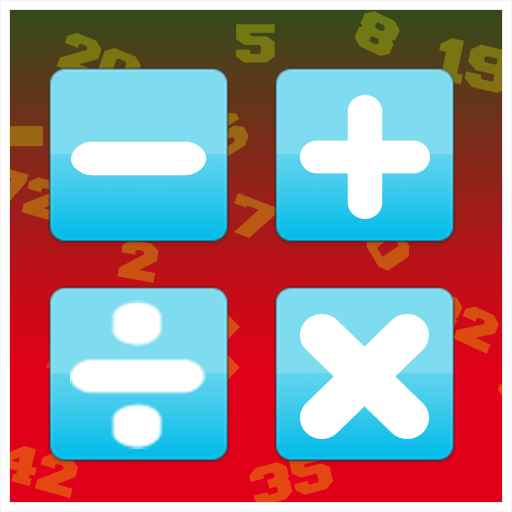## Elementary arithmetic Game## Elementary arithmetic Game

Description:

You have 60 seconds to complete a many equation as possible. In each equation, the elementary arithmetic is missing and you have to choose the right one based on the numbers and answer and complete the equation. When your 60 seconds end, your score is calculated based on the number of your correct and wrong answers. So be quick and do your best to correctly answer as many questions as you can.

Instructions:

Touch on mobile device or mouse on PC.

Favorite:
Play it online!
Game not working?
×
Elementary arithmetic Game is not working?
* You should use least 20 words.

## Elementary arithmetic Game Video Walkthrough

Description:

You have 60 seconds to complete a many equation as possible. In each equation, the elementary arithmetic is missing and you have to choose the right one based on the numbers and answer and complete the equation. When your 60 seconds end, your score is calculated based on the number of your correct and wrong answers. So be quick and do your best to correctly answer as many questions as you can.

Instructions:

Touch on mobile device or mouse on PC.